# PSEB 6th Class Maths Solutions Chapter 9 Understanding Elementary Shapes Ex 9.2

Punjab State Board PSEB 6th Class Maths Book Solutions Chapter 9 Understanding Elementary Shapes Ex 9.2 Textbook Exercise Questions and Answers.

## PSEB Solutions for Class 6 Maths Chapter 9 Understanding Elementary Shapes Ex 9.2

1. Classify the angles as acute, obtuse, right, straight or reflex angles: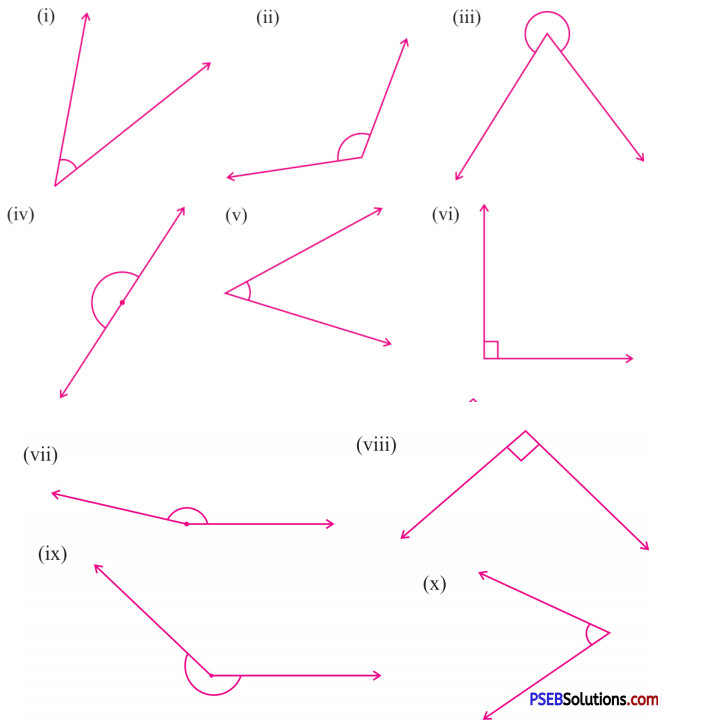Solution:
(i) Acute angle
(ii) Obtuse angle
(iii) Reflex angle
(iv) Straight angle
(v) Acute angle
(vi) Right angle
(vii) Obtuse angle
(viii) Right angle
(ix) Reflex angle
(x) Acute angle.2. Classify the angles:

Question (i)
80°
Solution:
80° is between 0° and 90°.
∴ It is an acute angle.

Question (ii)
172°
Solution:
172° is between 90° and 180°
∴ It is an obtuse angle.

Question (iii)
90°
Solution:
90° is a right angle.

Question (iv)

Solution:
0° is a zero angle.

Question (v)
179°
Solution:
179° is between 90° and 180°.
∴ It is an obtuse angle.Question (vi)
215°
Solution:
215° is between 180° and 360°.
∴ It is an reflex angle.

Question (vii)
360°
Solution:
360° is a complete angle.

Question (viii)
350°
Solution:
350° is between 180° and 360°.
∴ It is a reflex angle.

Question (ix)
15°
Solution:
15° is between 0° and 90°.
∴ It is an acute angle.

Question (x)
180°
Solution:
180° is a straight angle.3. Measure the following angles with protractor and write their measurement: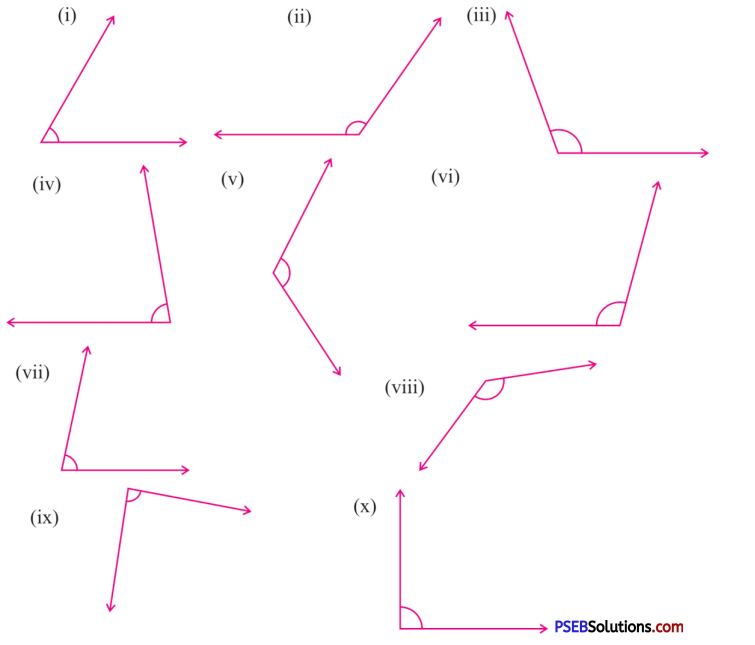Solution:
(i) 60°
(ii) 125°
(iii) 110°
(iv) 80°
(v) 120°
(vi) 105°
(vii) 80°
(viii) 135°
(ix) 88°
(x) 90°.

4. How many degrees are there in

Question (i)
Two right angles
Solution:
1 right angle = 90°
∴ Two right angles = 2 × 90°
= 180°Question (ii)
$$\frac {2}{3}$$ right angles
Solution:
1 right angle = 90°
∴ $$\frac {2}{3}$$ right angles = $$\frac {2}{3}$$ × 90°
= 2 × 30°
= 60°

Question (iii)
Four right angles?
Solution:
1 right angle = 90°
∴ Four right angles = 4 × 90°
= 360°

5. What fraction of a clockwise revolution does the hour hand of a clock turn through when it goes from:

Question (i)
3 to 9
Solution:
3 to 9 : Half or $$\frac {1}{2}$$

Question (ii)
5 to 8
Solution:
5 to 8 : Quarter or $$\frac {1}{4}$$

Question (iii)
10 to 4
Solution:
10 to 4 : Half or $$\frac {1}{2}$$Question (iv)
2 to 11
Solution:
2 to 11 : 3 Quarters or $$\frac {3}{4}$$

Question (v)
6 to 3
Solution:
6 to 3 : 3 Quarters or $$\frac {3}{4}$$

Question (vi)
2 to 7.
Solution:
2 to 7 : $$\frac {5}{12}$$

6. Find the number of right angles turned through by the hour hand of a dock when it goes from

Question (i)
5 to 8
Solution:
5 to 8 : 1 right angle

Question (ii)
1 to 7
Solution:
1 to 7 : 2 right angles

Question (iii)
4 to 10
Solution:
4 to 10 : 2 right anglesQuestion (iv)
9 to 12
Solution:
9 to 12 : 1 right angles

Question (v)
11 to 2
Solution:
11 to 2 : 1 right angles

Question (vi)
9 to 6
Solution:
9 to 6 : 3 right angles

Question (vii)
2 to 11
Solution:
2 to 11 : 3 right angles

Question (viii)
10 to 1
Solution:
10 to 1 : 1 right angles

Question (ix)
12 to 6
Solution:
12 to 6 : 2 right anglesQuestion (x)
5 to 2.
Solution:
5 to 2 : 3 right angles.

7. Where will be the hand of a clock stop if it starts at:

Question (i)
12 and make $$\frac {1}{4}$$ revolution clock-wise.
Solution:
For 1 revolution, the hour hand takes 12 hours.
For $$\frac {1}{4}$$ revolution, the hour hand takes $$\frac {1}{4}$$ × 12 hours = 3 hours.
If hour hand starts at 12 and make $$\frac {1}{4}$$ revolution clockwise it will stop at 3.

Question (ii)
2 and make $$\frac {1}{2}$$ revolution clock-wise.
Solution:
For 1 revolution, the hour hand takes 12 hours.
For $$\frac {1}{2}$$ revolution, the hour hand takes $$\frac {1}{2}$$ × 12 hours = 6 hours.
If hour hand starts at 2 and make $$\frac {1}{2}$$ revolution clockwise it will stop at 8.Question (iii)
5 and make $$\frac {1}{4}$$ revolution clock-wise.
Solution:
For 1 revolution, the hour hand takes 12 hours.
For $$\frac {1}{4}$$ revolution, the hour hand takes $$\frac {1}{4}$$ × 12 hours = 3 hours
If hour hand starts at 5 and make $$\frac {1}{4}$$ revolution clockwise it will stop at 8.

Question (iv)
5 and make $$\frac {3}{4}$$ revolution clock-wise.
Solution:
For 1 revolution, the hour hand takes 12 hours .
For $$\frac {3}{4}$$ revolution, the hour hand takes $$\frac {3}{4}$$ × 12 hours = 9 hours.
If hour hand starts at 5 and make $$\frac {3}{4}$$ revolution clockwise it will stop at 2.

8. What part of revolution have you turned through if you stand facing:

Question (i)
East and turn clockwise to North
Solution:
I turned through $$\frac {3}{4}$$ part of a revolution.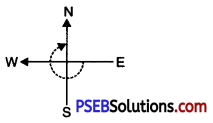Question (ii)
South and turn clockwise to North
Solution:
I turned through $$\frac {1}{2}$$ part of a revolution.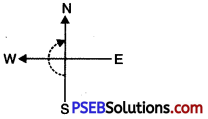Question (iii)
South and turn clockwise to East
Solution:
I turned through $$\frac {3}{4}$$ part of a revolution.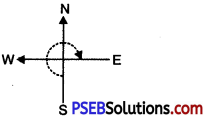Question (iv)
West and turn clockwise to East
Solution:
I turned through $$\frac {1}{2}$$ part of at revolution.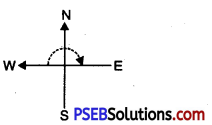9. Find the angle measure between the hands of the clock in each figure: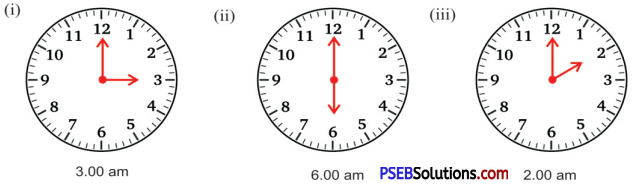Solution:
(i) Angle measure between the hands of the clock at 3.00 a.m.
= $$\frac {3}{12}$$ × 360° = 90°
(ii) Angle measure between the hands of the clock at 6.00 a.m.
= $$\frac {6}{12}$$ × 360° = 180°
(iii) Angle measure between the hands of the clock at 2.00 a.m.
= $$\frac {2}{12}$$ × 360° = 60°10. Draw the following angles by protractor:

Question (i)
(i) 40°
(ii) 75°
(iii) 105°
(iv) 90°
(v) 130°
Solution: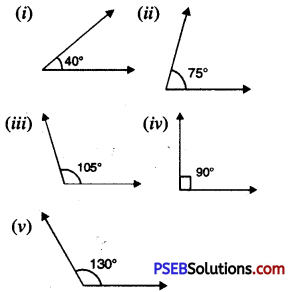11. State true or false:

Question (i)
The sum of two right angles is always a straight angle.
Solution:
TrueQuestion (ii)
The sum of two acute angles is always a reflex angle.
Solution:
False

Question (iiii)
The obtuse angle has measurement between 90° to 180°.
Solution:
True

Question (iv)
A complete revolution has four right angles.
Solution:
True

12. Fill in the blanks:

Question (i)
The angle which is greater than 0° and less than 90° is called ………….. .
Solution:
acute angleQuestion (ii)
The angle whose measurement equal to two right angle is …………….. .
Solution:
straight angle

Question (iii)
The angle between 90° and 180° is ……………. .
Solution:
obtuse angle.